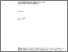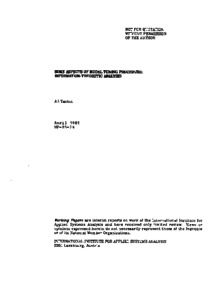Some Aspects of Model Tuning Procedure: Information-Theoretic Analysis

Yashin AI (1985). Some Aspects of Model Tuning Procedure: Information-Theoretic Analysis. IIASA Working Paper. IIASA, Laxenburg, Austria: WP-85-024Preview
Text
WP-85-024.pdf

Abstract

Computer or mathematical models are not exact representation of reality: lack of knowledge, technical restrictions and particular modeling goals make it necessary to approximate the real system in various ways. Nevertheless, the procedures by which the models are adjusted to observed data are often based on the assumptions that the real system has the same structure as the model and differs only in the values of certain parameters. These particular values usually should be included in the feasible set of the parameter values, and this fact, together with some additional conditions, usually provides the convergence property for many individual algorithms.

However, in reality all of these assumptions are generally false. Even if the structure of the system corresponds to the structure of the model, the real parameters values often do not belong to the presupposed feasible set. Moreover, mathematicians often consciously diminish this set in order to simplify the estimation algorithms. For instance they approximate the bounded compact set of parameter values by a set consisting of a finite number of points, thus increasing the chances that the real parameter values will be excluded.

It is therefore both remarkable and surprising to find that despite these false assumptions and approximations, the parameter estimation algorithms often still converge! The model resulting from this tuning procedure will of course not coincide with the real system. and this rises the natural question: how far is this computer model from reality?

When considering this question it is necessary to have some way of measuring the distance between individual models. One of measure of divergence was introduced by Bhattacharya; Kullback also formulated some measure of information distance. However these measures were not proper metrics. Baram and Sandell later introduced a modified version of Kullback measure, which have been shown to be a proper distance metric. They applied this approach to linear Gaussian systems and models; in this paper it is generalized to a wider class of systems....

Item Type: Monograph (IIASA Working Paper) World Population (POP) IIASA Import 15 Jan 2016 01:56 14 Mar 2018 09:00 http://pure.iiasa.ac.at/2676View Item